# Difference between revisions of "SOCR EduMaterials AnalysisActivities TwoIndepT"

## SOCR Analysis - Two Independent Sample T-Test Background

Given two groups of data, not necessarily with same sample size, we'd like to test the null hypothesis that the two groups have equal mean. This is a parametric test.

## Two Independent Sample T-Test Example (Pooled Sample Variance)

Go to the SOCR Two Independent Sample T-Test Applet. We will demonstrate Two Independent T-Test with a SOCR built-in example. This example is based on the data taken from "Mathematical Statistics and Data Analysis" by John A. Rice, Second Edition, Ducbury Press, 1995. (Page 390)

Here's the steps of the activity:

1. Click on Two Independent Sample T-Test (Poored) at the left panel's combo box.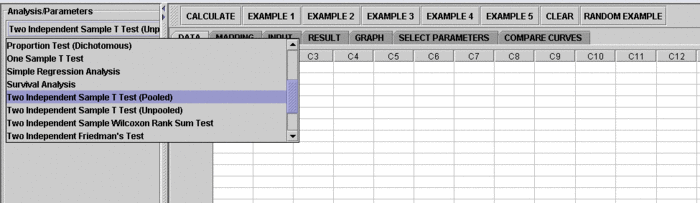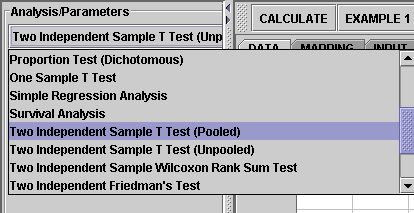On the right panel, first click on EXAMPLE 1 and next click on "Data" to retreive the demonstrated example. This is the example we'll be looking at.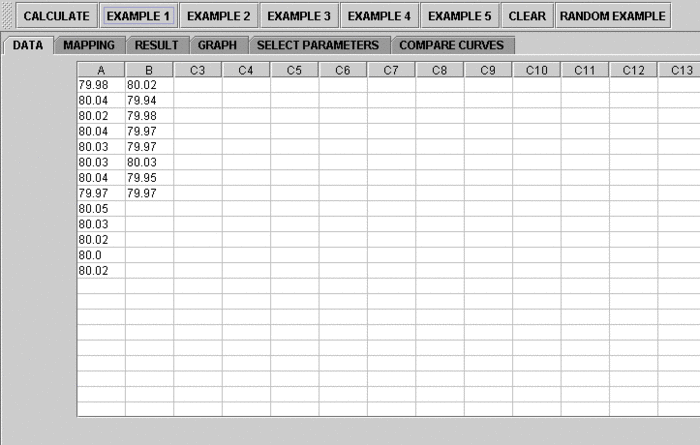2. Click on the "Mapping" button to get to the "Mapping" panel. Click on "ADD" under VARIABLE 1 and to add A, and click on ADD under VARIABLE 2 to add B. This will add the variables for Two Independent Sample T-Test. We care about the difference, so which on goes to VARIABLE 1 or VARIALBE 2 is arbitrary. Here, the computer will set difference = Variable 2 - Variable 1. If you like the subtraction to go the other way around, simple assign VARIABLE 1 and VARIABLE 2 in the opposite way.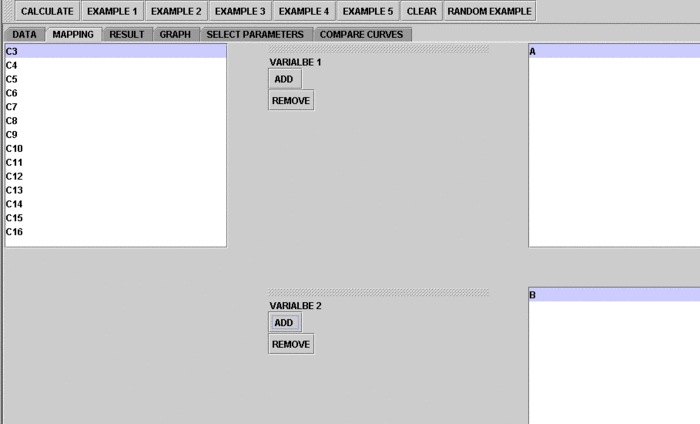3. Click on "Calculate" then click on "Result" to see the results.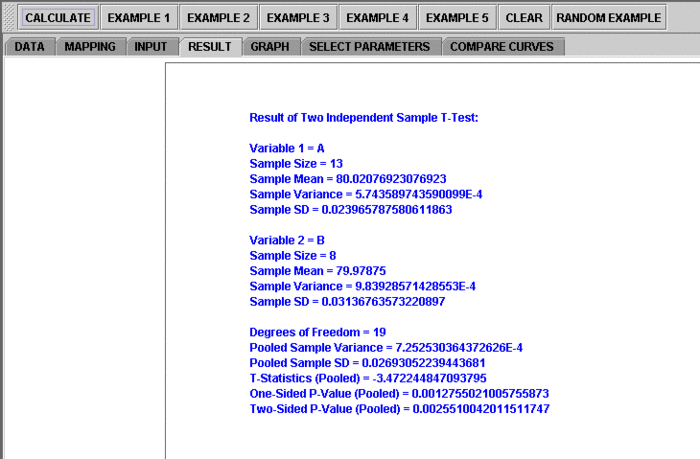Questions for students: By looking at the test results, what do you think about the data? What can you say about the two different shoe materials?